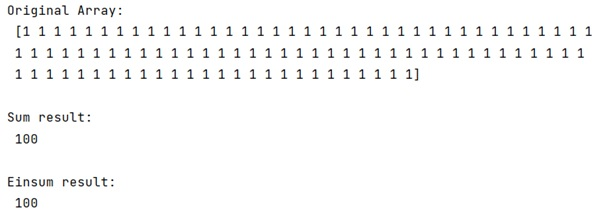# Why is numpy's einsum faster than numpy's built in functions?

Learn, why is numpy's einsum faster than numpy's built in functions?
Submitted by Pranit Sharma, on January 18, 2023

NumPy is an abbreviated form of Numerical Python. It is used for different types of scientific operations in python. Numpy is a vast library in python which is used for almost every kind of scientific or mathematical operation. It is itself an array which is a collection of various methods and functions for processing the arrays.

## numpy's einsum vs numpy's built in functions

The einsum() seems to be at least twice as fast for np.inner, np.outer, np.kron, and np.sum regardless of axes selection. The primary exception is np.dot as it calls DGEMM from a BLAS library.

So, the question arises thats why is np.einsum() faster that other NumPy functions that are equivalent?

The logical fact that can be given on this topic is that einsum() is new, and is presumably trying to be better about cache alignment and other memory access issues, while many of the older NumPy functions focus on easily portable implementation over a heavily optimized one.

But also, not everything about einsum() is better than other NumPy methods. The sum() uses a more appropriate accumulator for arrays.

For example, sum() is more careful about checking the type of input and using an appropriate accumulator.

## Python code to demonstrate why numpy's einsum faster than numpy's built in functions

```# Import numpy
import numpy as np

# Creating a numpy array
arr = np.ones(100, dtype=np.uint8)

# Display original array
print("Original Array:\n",arr,"\n")

# Using sum method
res = arr.sum()

# Display sum result
print("Sum result:\n",res,"\n")

# Using einsum
res = np.einsum('i->', arr)

# Display einsum result
print("Einsum result:\n",res)
```

Output:What's New (MCQs)

Top Interview Coding Problems/Challenges!

IncludeHelp's Blogs

Languages: » C » C++ » C++ STL » Java » Data Structure » C#.Net » Android » Kotlin » SQL
Web Technologies: » PHP » Python » JavaScript » CSS » Ajax » Node.js » Web programming/HTML
Solved programs: » C » C++ » DS » Java » C#
Aptitude que. & ans.: » C » C++ » Java » DBMS
Interview que. & ans.: » C » Embedded C » Java » SEO » HR
CS Subjects: » CS Basics » O.S. » Networks » DBMS » Embedded Systems » Cloud Computing
» Machine learning » CS Organizations » Linux » DOS
More: » Articles » Puzzles » News/Updates